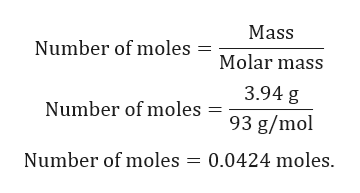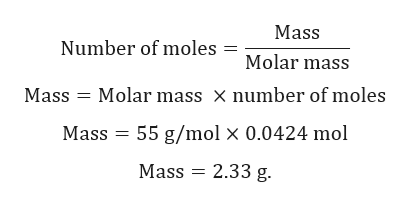# How many GRAMS of manganese(II) are present in 3.94 grams of manganese(II) fluoride, MnF2 ?

Question
34 views

How many GRAMS of manganese(II) are present in 3.94 grams of manganese(II) fluorideMnF2 ?

check_circle

Step 1

Given:

Mass of MnF2 = 3.94 g.

Molar mass of MnF2 = 93 g/mol.

Molar mass of Mn = 55 g/mol.

Step 2

Calculation for number of moles of MnF2:help_outlineImage TranscriptioncloseMass Number of moles = Molar mass 3.94 g Number of moles = 93 g/mol Number of moles 0.0424 moles fullscreen
Step 3

1 mole of MnF2 contains 1 mole of Mn.

0.0424 mole of MnF2 contains 0.042...help_outlineImage TranscriptioncloseMass Number of moles = Molar mass Molar mass x number of moles Mass Mass 55 g/mol x 0.0424 mol Mass 2.33 g fullscreen

### Want to see the full answer?

See Solution

#### Want to see this answer and more?

Solutions are written by subject experts who are available 24/7. Questions are typically answered within 1 hour.*

See Solution
*Response times may vary by subject and question.
Tagged in

### General Chemistry